# Multi Dimensional Recurrent Networks

Tensorflow Implementation of the model described in Alex Graves' paper https://arxiv.org/pdf/0705.2011.pdf.

## What is MD LSTM?

Basically a LSTM that is multidirectional, for example, that can operate on a 2D grid. Here's a figure describing the way it works:

## How to get started?

```git clone [email protected]:philipperemy/tensorflow-multi-dimensional-lstm.git
cd tensorflow-multi-dimensional-lstm

# create a new virtual python environment
virtualenv -p python3 venv
source venv/bin/activate
pip install -r requirements.txt

# usage: trainer.py [-h] --model_type {MD_LSTM,HORIZONTAL_SD_LSTM,SNAKE_SD_LSTM}
python trainer.py --model_type MD_LSTM
python trainer.py --model_type HORIZONTAL_SD_LSTM
python trainer.py --model_type SNAKE_SD_LSTM
```

The random diagonal task consists in initializing a matrix with values very close to 0 except two which are set to 1. Those two values are on a straight line parallel to the diagonal of the matrix. The idea is to predict where those two values are. Here are some examples:

``````____________
|          |
|x         |
| x        |
|          |
|__________|

____________
|          |
|          |
|     x    |
|      x   |
|__________|

____________
|          |
| x        |
|  x       |
|          |
|__________|

``````

A model performing on this task is considered as successful if it can correctly predict the second x (it's impossible to predict the first x).

• A simple recurrent model going vertical or horizontal cannot predict any locations of x. This model is called `HORIZONTAL_SD_LSTM`. It should perform the worst.
• If the matrix is flattened as one single vector, then the first location of x still cannot be predicted. However, a recurrent model should understand that the second x always comes after the first x (width+1 steps). (Model is `SNAKE_SD_LSTM`).
• When predicting the second location of x, a MD recurrent model has a full view of the TOP LEFT corner. In that case, it should understand that when the first x is in the bottom right of its window, the second x will be next on the diagonal axis. Of course the first location x still cannot be predicted at all with this MD model.

After training on this task for 8x8 matrices, the losses look like this:

Overall loss of the random diagonal task (loss applied on all the elements of the inputs)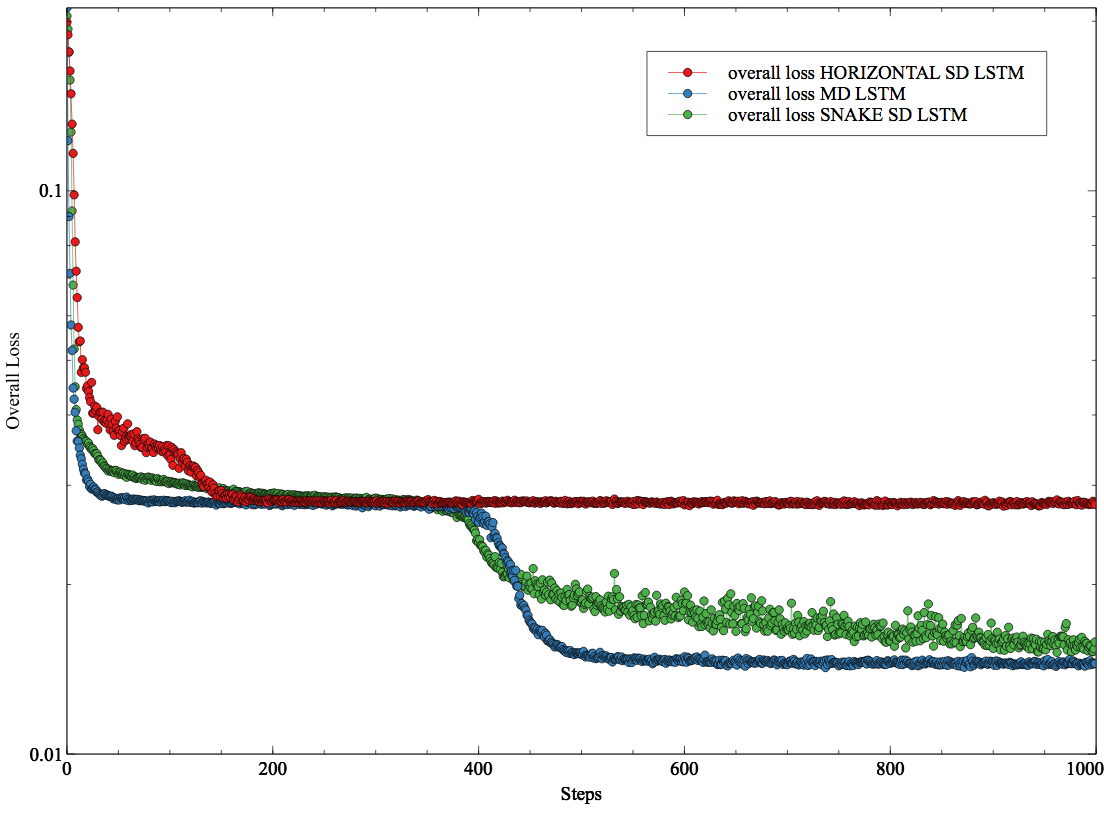Overall loss of the random diagonal task (loss applied only on the location of the second x)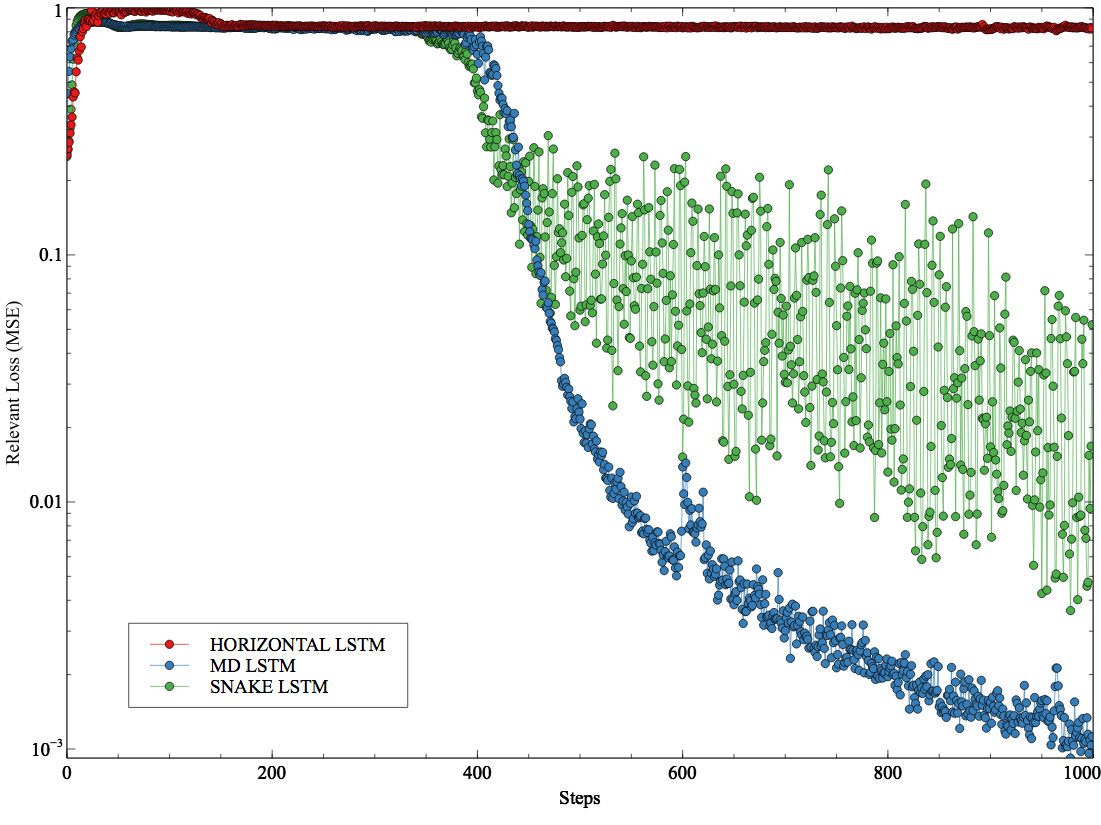No surprise that MD LSTM performs the best here. It has direct connections between the grid cell that contains the first x and the second x (2 connections). The snake LSTM has width+1 = 9 steps between the two x. As expected, the vertical LSTM does not learn anything apart from outputting values very close to 0.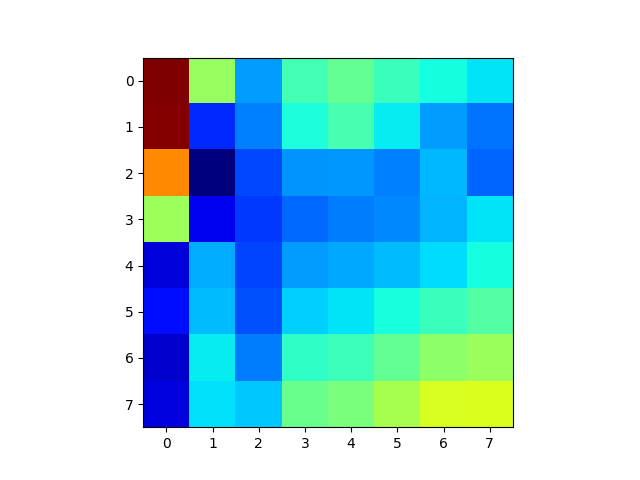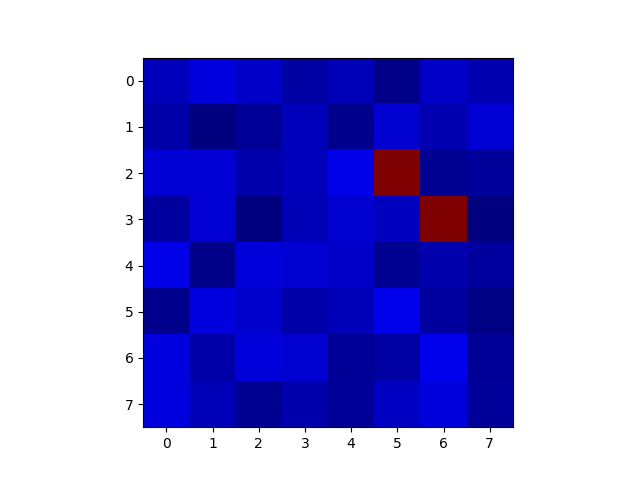MD LSTM predictions (left) and ground truth (right) before training (predictions are all random).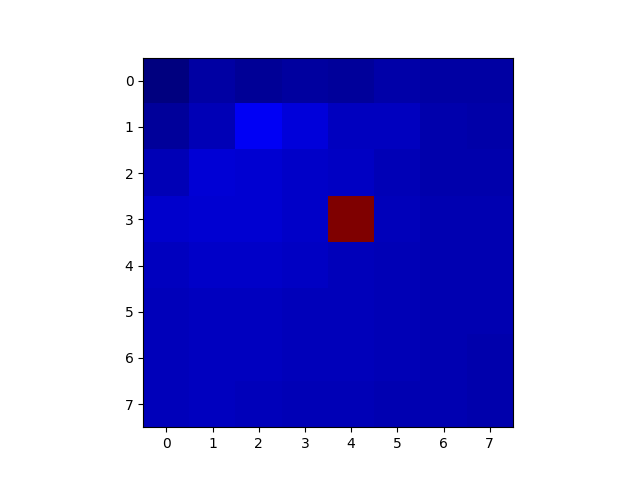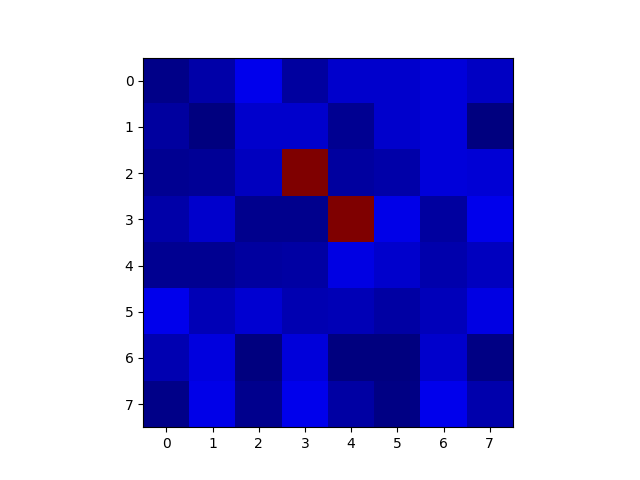MD LSTM predictions (left) and ground truth (right) after training. As expected, the MD LSTM can only predict the second x and not the first one. That means the task is correctly predicted.

## Limitations

• I could test it successfully with 32x32 matrices but the implementation is far from being well optimised.
• This implementation can become numerically unstable quite easily.
• I've noticed that inputs should be != 0. Otherwise some gradients are nan. So consider `inputs += eps` in case.
• It's hard to use in Keras. This implementation is in pure tensorflow.
• It runs on a GPU but the code is not optimized at all so I would say it's equally fast (CPU vs GPU).

Welcome!

## Special Thanks

• A big thank you to Mosnoi Ion who provided the first skeleton of this MD LSTM.

Get A Weekly Email With Trending Projects For These Topics
No Spam. Unsubscribe easily at any time.
jupyter-notebook (6,203
lstm (266